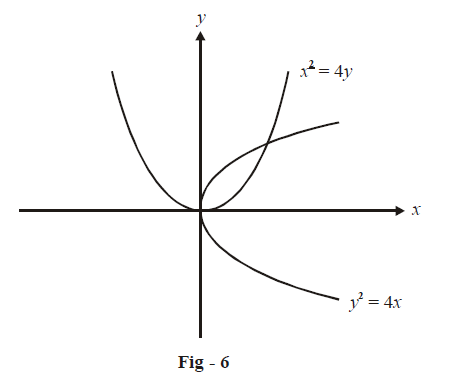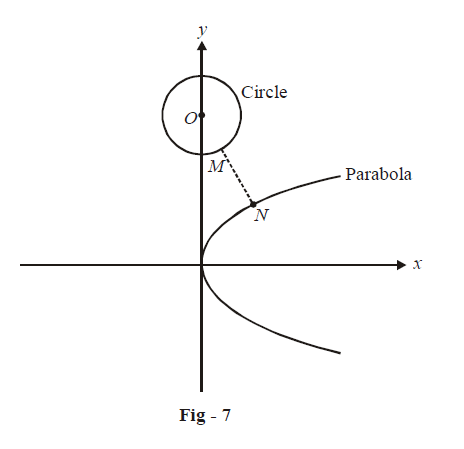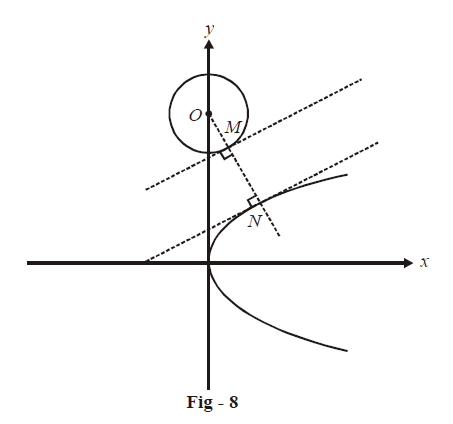# Examples on Tangents and Normals Set 4

Go back to  'Applications of Derivatives'

### Example – 9

Find the angle of intersection between $${y^2} = 4x\,\,{\rm{and}}\,\,{x^2} = 4y$$.

Solution:There are two points of intersection, which can be obtained by simultaneously solving the equations for the two curves.

\begin{align}&{y^2} = 4x\,\,{\rm{and}}\,\,{x^2} = 4y\\\\ \Rightarrow \qquad \quad &{y^4} = 16{x^2} = 64y\\\\ \Rightarrow \qquad \quad &y\left( {{y^3} - 64} \right) = 0\\\\\Rightarrow \qquad \quad &y = 0,\,4\\\\ \Rightarrow \qquad \quad &\!\!\!\text{The points of intersection are (0, 0) and (4, 4). Let} \; m_{T_1}\; \rm{and}\; m_{T_2} \\&\!\!\text {represent the slopes of tangents to}\;x^2\,=\,4y\; \rm{and}\; y^2 = 4x \;\text {respectively.}\end{align}

\begin{align}\rm{\bf{At (0, 0):}} \qquad \qquad \qquad &{m_{{T_1}}} = {\left. {\frac{{dy}}{{dx}}} \right|_{x = 0}} = \frac{x}{2} = 0\\\\&{m_{{T_2}}} = {\left. {\frac{{dy}}{{dx}}} \right|_{x = 0}} = \frac{2}{y} = \infty\\\\\Rightarrow \qquad &\text{The angle between these two tangents is obviously 90° which is visually}\\&\text{clear from Fig – 6.}\end{align}

\begin{align}\rm{\bf{At (4, 4):}}\qquad \qquad \qquad &{m_{{T_1}}}{\left. { = \frac{{dy}}{{dx}}} \right|_{x = 4}} = \frac{x}{2} = 2\\\\&{m_{{T_2}}}{\left. { = \frac{{dy}}{{dx}}} \right|_{x = 4}} = \frac{2}{y} = \frac{1}{2}\end{align}

The angle of intersection is:

$\theta ={{\tan }^{-1}}\left( \frac{2-{}^{1}\!\!\diagup\!\!{}_{2}\;}{1+2\times {}^{1}\!\!\diagup\!\!{}_{2}\;} \right)={{\tan }^{-1}}\left( \frac{3}{4} \right)$

Therefore, the two curves intersect in two points, once at $${{90}^{\circ }}$$ and once at  \begin{align}{\tan ^{ - 1}}\left( {\frac{3}{4}} \right)\end{align}

Example – 10

Find the shortest distance between two points, one of which lies on the curve $${y^2} = 4ax,$$  and the other on the circle $${x^2} + {y^2} - 24ay + 128{a^2} = 0$$

Solution: Notice that the circle’s equation can be written equivalently as

${\left( {x - 0} \right)^2} + {\left( {y - 12a} \right)^2} = {\left( {4a} \right)^2}$

so that its centre is (0, 12a) and radius is 4a.Let MN represent the shortest distance between the circle and the ellipse. Since the point N on the ellipse is nearest to the circle, it will also be nearest to the centre of the circle O, from amongst all the other points on the ellipse. Hence, to determine MN, we may equivalently find the shortest distance between the circle’s centre and any point on the ellipse.

Now, from Fig-8’s geometry, notice a very important fact. The tangent drawn at M must be perpendicular to ON, or equivalently, ON must be a normal to the ellipse. Only then will N be the closest point on the ellipse from O. (Convince yourself that this should be true)We take an arbitrary point on the parabola as $$(at{^2},{\rm{ }}2at)$$. We will write the normal to the parabola at this point and make this normal pass through the point O.

\begin{align}&{y^2} = 4ax\\\\ \Rightarrow \qquad &2y\frac{{dy}}{{dx}} = 4a\\\\\Rightarrow \qquad &\frac{{dy}}{{dx}} = \frac{{2a}}{y}\\\\&{m_N}\left( {at\left( {a{t^2},2at} \right)} \right) = {\left. {\frac{{ - dx}}{{dy}}} \right|_{\left( {a{t^2}, \, 2at} \right)}} = - t \end{align}

\begin{align}\rm{\bf{Equation \;\;of\;\; normal:}}\qquad \qquad \qquad &y - 2at = - t\left( {x - a{t^2}} \right)\\\Rightarrow \qquad &tx + y = 2at + a{t^3} \qquad \qquad \dots \rm{(i)}\end{align}

So that this normal passes through O, the co-ordinates of O(0, 12a) must satisfy (i)

\begin{align} \Rightarrow \qquad &12a = 2at + a{t^3}\\\\ \Rightarrow \qquad &2t + {t^3} = 12\\\\ \Rightarrow \qquad &t = 2\;\;\;\;\;\;\;\; \rm{(verify)} \end{align}

We therefore get the co-ordinates of N as (4a, 4a).

\begin{align}\text{Hence,} \qquad \qquad \qquad &ON = \sqrt {{{\left( {4a - 0} \right)}^2} + {{\left( {4a - 12a} \right)}^2}} = \sqrt {80{a^2}} = 4\sqrt 5 a\\\\\Rightarrow \qquad &MN = 4\sqrt 5 a - 4a = \,\,\,4\left( {\sqrt 5 - 1} \right)a \end{align}

## TRY YOURSELF - I

Q. 1 Find the equation of the tangent to the curve \begin{align}{\left( {\frac{x}{a}} \right)^n} + {\left( {\frac{y}{b}} \right)^n} = 20\end{align} at the point (a, b).

Q. 2 If l1 and l2 are the lengths of the perpendiculars drawn from the origin on the gangent and normal to the curve \begin{align}{x^{2/3}} + {y^{2/3}} = {a^{2/3}}\end{align} respectively at an arbitrary point, find the value of \begin{align}4l_1^2 + l_2^2\end{align} in terms of a.

Q. 3 Find the sum of the intercepts made on the coordinate axes by any tangent to the curve $$\sqrt x + \sqrt y = \sqrt a$$

Q. 4 Find the angle at which the curves $$2{y^2} = {x^3}\;{\rm{and}}\;{y^2} = 32x$$ intersect at the origin.

Q. 5 If the tangent at $$\left( {{x_1},{y_1}} \right)$$ to $${x^3} + {y^3} = {a^3}$$ meets the curve again at $$\left( {{x_2},{y_2}} \right),$$ find the value of \begin{align}\frac{{{x^2}}}{{{x_1}}} + \frac{{{y_2}}}{{{y_1}}}.\end{align}

Q. 6 If  $$ax + by = 1$$ is a normal to the parabola $${y^2} = 4cx$$, find the value of \begin{align}\frac{{{b^2} - c{a^3}}}{{a{b^3}c}}.\end{align}

Q. 7 Do the curves \begin{align}xy = 4\;{\rm{and}}\;{x^2} + {y^2} = 8\end{align} touch each other or intersect?

Q. 8 Find the equations of tangents drawn from (1, 2) to be curve \begin{align}{y^2} - 2{x^3} - 4y + 8 = 0\end{align}